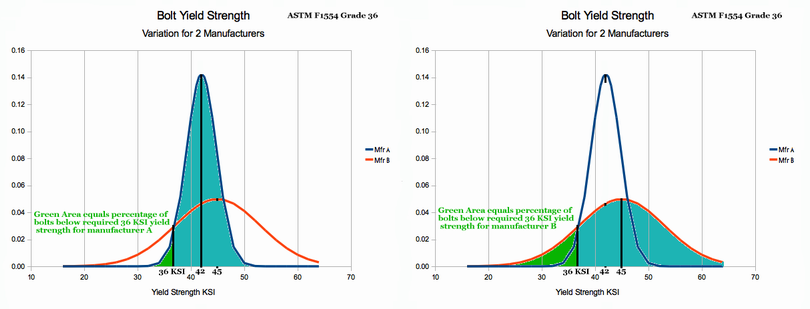# St. Paul's Statistics Introduction: Homework Problem 1:

### For the Salvation of Many by Paul F. Watson

Ch2 Homework Problem 1: Using the four criteria above:

1. Decide whether or not the binomial distribution can be used to analyse the toss of 10 coins that was discussed above (yes or no)
2. Write a brief list of steps that justify your answer.

To solve this problem, we need to compare the problem description to each of the four criteria shown below. The binomial method can only be used if the problem matches all four criteria.

Criteria for Using the Binomial Distribution
When the following four conditions are ALL true, the Binomial Distribution can be confidently used.

• The number of trials is known and (usually) less than 12
• Each trial has a binary result (i.e. a torpedo hits, or does not hit. A student fails or does not fail, A student eats liver, or does not)
• The probability of 'success' is known (e.g. .15%, 35%, 50% etc.)
• The success of each trial is independent of the other trials. This means that after a torpedo hit, the next torpedo is neither more, nor less likely to hit.)

1. We are talking about 10 items. Definitely we know the number of trials and its less than 12. GOOD
2. I guess that the intent of the problem is to analyse the number of "heads" during a coin toss. Each individual coin will either be "heads" or "not heads". Yes, the result is binary. GOOD
3. Most coins I ever saw came up heads half the time. That is 50%. GOOD
4. I do not believe that one coin is influenced by the fall of another. Coins just aren't that smart. GOOD
All four Binomial use criteria have been reviewed against the problem. Yes, we can use the binomial method for this problem.

Use the back arrow on the browser to return to Chapter 2.

Contact the author paul-watson@sbcglobal.net by e-mail.